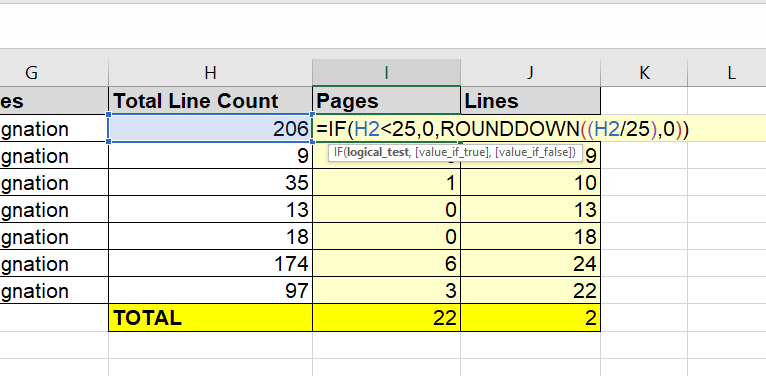top of pageSearch

# Calculating the total page and line count for deposition designations

You can use the Excel file linked to at the bottom of this post to automatically calculate how many pages and lines are in a single deposition designation, and multiple designations.

The beginning and ending pages and lines for each range of testimony are entered in columns B through E. Column H has a formula which computes the total number of lines in a single range of testimony:

=IF(B2=D2,(E2-C2)+1,(E2-C2)+((D2-B2)*25)+1)If the designation is only on a single page, it simply subtracts the line number in column C from column E. If this is not the case, it multiplies the number of pages by 25, and adds them to the difference between E and C to get the line total.

Column I contains the formula that divides the line count by 25, and rounds down the result to the nearest whole number, unless there are less than 25 lines:

=IF(H2<25,0,ROUNDDOWN((H2/25),0))The formula in column J: =IF(I2=0,H2,H2-(I2*25))

. . .subtracts the line count for the rounded down result given in column I from the total line count, unless there is less than 1 page, in which case it returns the total line count from column H.On the TOTAL row, the formula in cell I9 not only adds up the page total listed above in column I, but also gives the sum of lines in column J divided by 25, rounded down to the nearest whole number. This gives the total page count for all the designations given in columns B to E on the above rows.

=SUM(I2:I8)+(ROUNDDOWN((SUM(J2:J8))/25,0))Finally, the formula in J9 subtracts [the total line count divided by 25 and rounded down to the nearest whole number, but then multiplied by 25 - to get a number divisible by 25] FROM the total line count, in order to get the number of lines left over when the lines adding up to full pages are counted .

=SUM(J2:J8)-(ROUNDDOWN((SUM(J2:J8))/25,0)*25)Deposition Designations v3
.xlsx